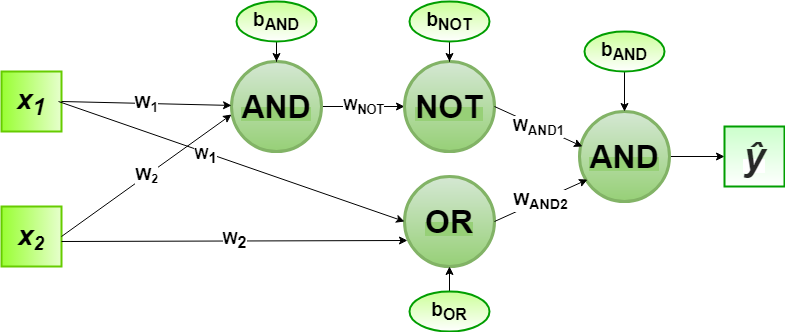# Implementation of Perceptron Algorithm for XOR Logic Gate with 2-bit Binary Input

In the field of Machine Learning, the Perceptron is a Supervised Learning Algorithm for binary classifiers. The Perceptron Model implements the following function:For a particular choice of the weight vectorand bias parameter, the model predicts outputfor the corresponding input vector.

XOR logical function truth table for 2-bit binary variables, i.e, the input vectorand the corresponding output0 0 0
0 1 1
1 0 1
1 1 0

We can observe that,Designing the Perceptron Network:

1. Step1: Now for the corresponding weight vectorof the input vectorto the AND and OR node, the associated Perceptron Function can be defined as:2. Step2: The outputfrom the AND node will be inputed to the NOT node with weightand the associated Perceptron Function can be defined as:3. Step3: The outputfrom the OR node and the outputfrom NOT node as mentioned in Step2 will be inputed to the AND node with weight. Then the corresponding outputis the final output of the XOR logic function. The associated Perceptron Function can be defined as:For the implementation, the weight parameters are considered to beand the bias parameters are.

Python Implementation:

 # importing Python library  import numpy as np     # define Unit Step Function  def unitStep(v):      if v >= 0:          return 1     else:          return 0    # design Perceptron Model  def perceptronModel(x, w, b):      v = np.dot(w, x) + b      y = unitStep(v)      return y     # NOT Logic Function  # wNOT = -1, bNOT = 0.5  def NOT_logicFunction(x):      wNOT = -1     bNOT = 0.5     return perceptronModel(x, wNOT, bNOT)     # AND Logic Function  # here w1 = wAND1 = 1,   # w2 = wAND2 = 1, bAND = -1.5  def AND_logicFunction(x):      w = np.array([1, 1])      bAND = -1.5     return perceptronModel(x, w, bAND)     # OR Logic Function  # w1 = 1, w2 = 1, bOR = -0.5  def OR_logicFunction(x):      w = np.array([1, 1])      bOR = -0.5     return perceptronModel(x, w, bOR)     # XOR Logic Function  # with AND, OR and NOT    # function calls in sequence  def XOR_logicFunction(x):      y1 = AND_logicFunction(x)      y2 = OR_logicFunction(x)      y3 = NOT_logicFunction(y1)      final_x = np.array([y2, y3])      finalOutput = AND_logicFunction(final_x)      return finalOutput     # testing the Perceptron Model  test1 = np.array([0, 1])  test2 = np.array([1, 1])  test3 = np.array([0, 0])  test4 = np.array([1, 0])     print("XOR({}, {}) = {}".format(0, 1, XOR_logicFunction(test1)))  print("XOR({}, {}) = {}".format(1, 1, XOR_logicFunction(test2)))  print("XOR({}, {}) = {}".format(0, 0, XOR_logicFunction(test3)))  print("XOR({}, {}) = {}".format(1, 0, XOR_logicFunction(test4)))

Output:

XOR(0, 1) = 1
XOR(1, 1) = 0
XOR(0, 0) = 0
XOR(1, 0) = 1


Here, the model predicted output () for each of the test inputs are exactly matched with the XOR logic gate conventional output () according to the truth table.
Hence, it is verified that the perceptron algorithm for XOR logic gate is correctly implemented.

Attention geek! Strengthen your foundations with the Python Programming Foundation Course and learn the basics.

To begin with, your interview preparations Enhance your Data Structures concepts with the Python DS Course.

My Personal Notes arrow_drop_upCheck out this Author's contributed articles.

If you like GeeksforGeeks and would like to contribute, you can also write an article using contribute.geeksforgeeks.org or mail your article to contribute@geeksforgeeks.org. See your article appearing on the GeeksforGeeks main page and help other Geeks.

Please Improve this article if you find anything incorrect by clicking on the "Improve Article" button below.

Improved By : Akanksha_Rai